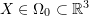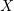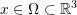### Balance Equations: Eulerian and Lagrangian Formulations

The term Lagrangian Formulation is usually used to describe a balance equation written as a function of the coordinates in the reference coordinate system, while the term Eulerian Formulation is usually used to describe an equation written as a function of the coordinates in the deformed coordinate system. In a Lagrangian formulation, the coordinates of a vectorcorrespond to a material point and are fixed in time, so differential equations written in terms ofdescribe the material rates of change of the different variables appearing in the differential equation. In an Eulerian formulation, quantities are written as a function of the spatial coordinates of the vector, thus, the quantities appearing in the differential equation point to a position in space rather than a material point. Traditionally, equations for solids usually use the Lagrangian formulations while those for fluids use the Eulerian formulations.